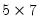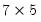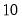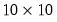Command Reference : Matrix Language Reference

 @transpose
Transpose of a matrix object.
Syntax: @transpose(m)
m: matrix, vector, rowvector, sym
Return: matrix, rowvector, vector, sym
The result is a matrix object with the number of rows and columns and the element indices reversed from the original matrix. This function is an identity function for a sym, since a sym by definition is equal to its transpose.
Examples
matrix m1 = @mnrnd(5, 7)
matrix m1t = @transpose(m1)
creates amatrix M1 filled with normal random variables, and thematrix transpose.
vector v1 = @mnrnd(10)
matrix m2 = v1 * @transpose(v1)
matrix m3 = @outer(v1, v1)
creates theelement vector V1 and forms theouter product matrices M2 and M3 both directly, and using the @outer function.
Note that the transpose of matrix objects may also be obtained using the “@t” object data member function, as in
matrix m2a = v1 * v1.@t
Cross-references